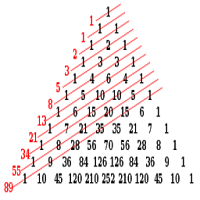Fibonacci Sequence Number Generator# Fibonacci Sequence Number Generator

## This generator will help you find the exact number in the sequence of Fibonacci.

App Size: 1.7M
Release Date: Apr 8, 2018
Price: Free
Price
Free
Size
1.7M

The Fibonacci Sequence is used to calculate retracement level's in the stock market. It is a series of numbers in which each number is the sum of previous two numbers. The Fibonacci Sequence starts off from 0 and 1 and goes to 1, 1, 2, 3, 5, 8, etc.

This generator will calculate the number you wish to generate. For Example the 5th number in the sequence is 3, so you will enter the number 5 and the generator will generate 3. Simply, you do not need to do the manual calculation.

What's new:

- Bug Fixes
- Friendly & Simple UI